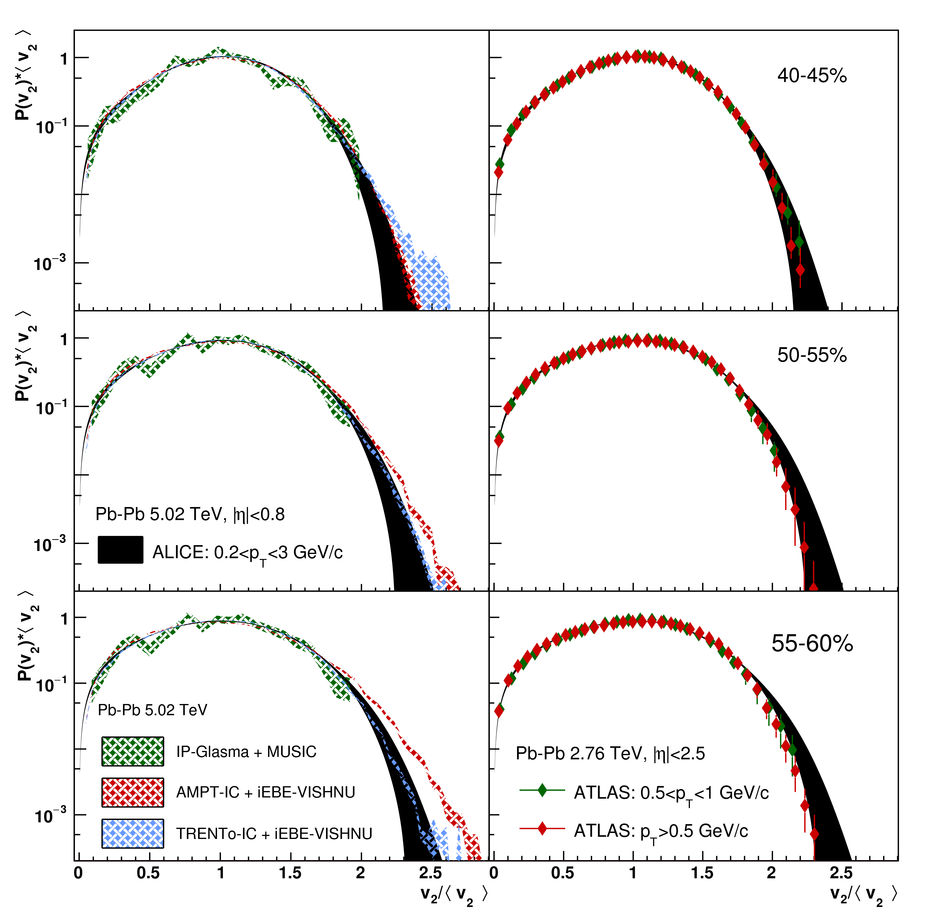Energy dependence and fluctuations of anisotropic flow in Pb-Pb collisions at $\mathbf{\sqrt{{\textit s}_\text{NN}}} = \mathbf{5.02}$ and $\mathbf{2.76}$ TeV

Measurements of anisotropic flow coefficients with two- and multi-particle cumulants for inclusive charged particles in Pb-Pb collisions at $\sqrt{{\textit s}_\text{NN}} = 5.02$ and 2.76 TeV are reported in the pseudorapidity range $|\eta| <~ 0.8$ and transverse momentum $0.2 <~ p_\text{T} <~ 50$ GeV/$c$. The full data sample collected by the ALICE detector in 2015 (2010), corresponding to an integrated luminosity of 12.7 (2.0) $\mu$b$^{-1}$ in the centrality range 0-80%, is analysed. Flow coefficients up to the sixth flow harmonic ($v_6$) are reported and a detailed comparison among results at the two energies is carried out. The $p_\text{T}$ dependence of anisotropic flow coefficients and its evolution with respect to centrality and harmonic number $n$ are investigated. An approximate power-law scaling of the form $v_n(p_\text{T}) \sim p_\text{T}^{n/3}$ is observed for all flow harmonics at low $p_\text{T}$ ($0.2 <~ p_\text{T} <~ 3$ GeV/$c$). At the same time, the ratios $v_n/v_m^{n/m}$ are observed to be essentially independent of $p_\text{T}$ for most centralities up to about $p_\text{T} = 10$ GeV/$c$. Analysing the differences among higher-order cumulants of elliptic flow ($v_2$), which have different sensitivities to flow fluctuations, a measurement of the standardised skewness of the event-by-event $v_2$ distribution $P(v_2)$ is reported and constraints on its higher moments are provided. The Elliptic Power distribution is used to parametrise $P(v_2)$, extracting its parameters from fits to cumulants. The measurements are compared to different model predictions in order to discriminate among initial-state models and to constrain the temperature dependence of the shear viscosity to entropy-density ratio.

Figures

Figure 1

 Anisotropic flow coefficients $v_n$ of inclusive charged particles as a function of centrality, for the two-particle (denoted with $|\Delta\eta|>1$) and four-particle cumulant methods. Measurements for Pb--Pb collisions at $\sqrt{{\textit s}_\text{NN}} = 5.02$ (2.76) TeV are shown by solid (open) markers.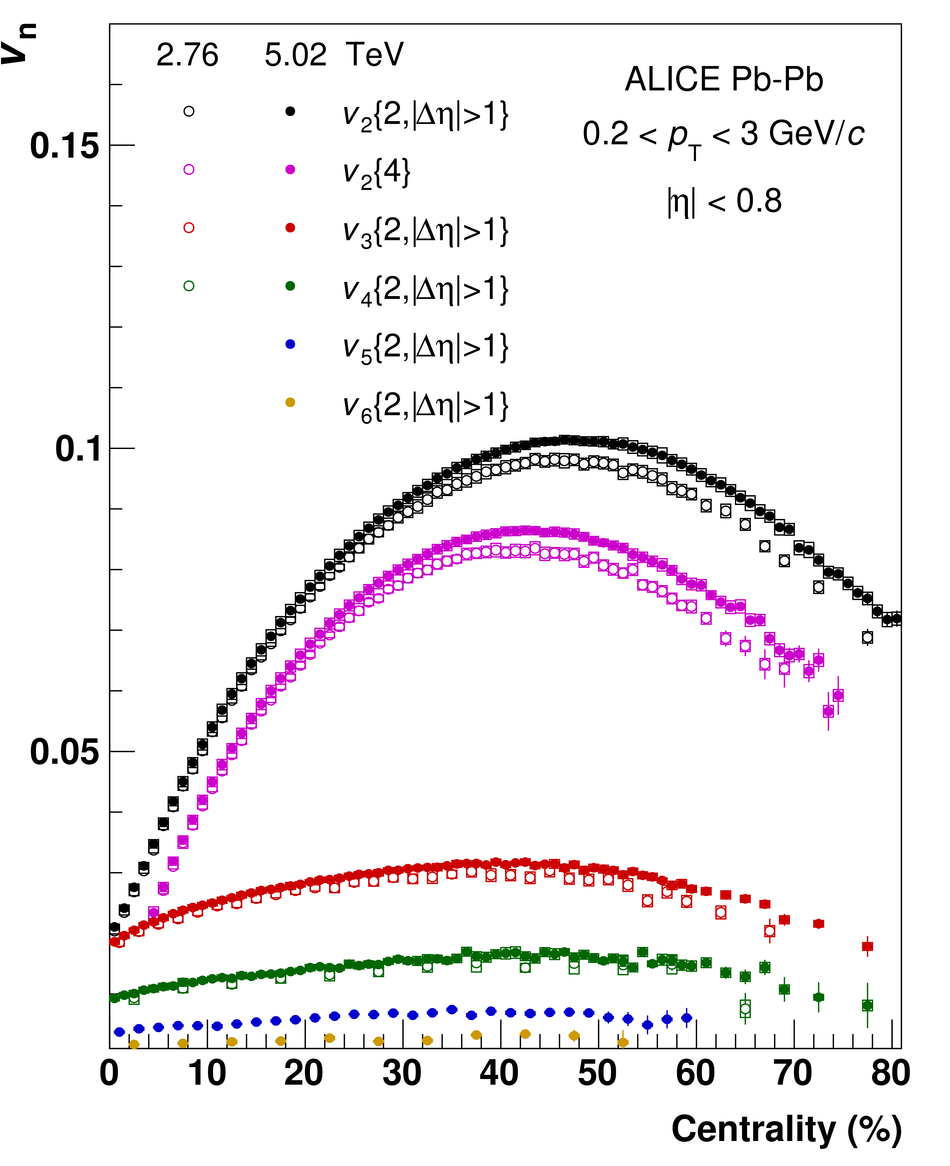Figure 2

 Ratios of anisotropic flow coefficients $v_n$ of inclusive charged particles between Pb--Pb collisions at $\sqrt{{\textit s}_\text{NN}} = 5.02$ and 2.76 TeV, as a function of centrality. Hydrodynamic calculations employing different $\eta/s(T)$ parametrizations are shown for comparison.Figure 3

 Anisotropic flow coefficients $v_n(p_\text{T})$ of inclusive charged particles in different centrality classes, measured with two-particle (denoted with $|\Delta\eta|>1$) and four-particle cumulant methods. Measurements for Pb--Pb collisions at $\sqrt{{\textit s}_\text{NN}} = 5.02$ (2.76) TeV are shown by solid (open) markers. Dashed lines are fits with a power-law function $v_n(p_\text{T}) = \text{A} \, p_\text{T}^{n/3}$, with A as free parameter.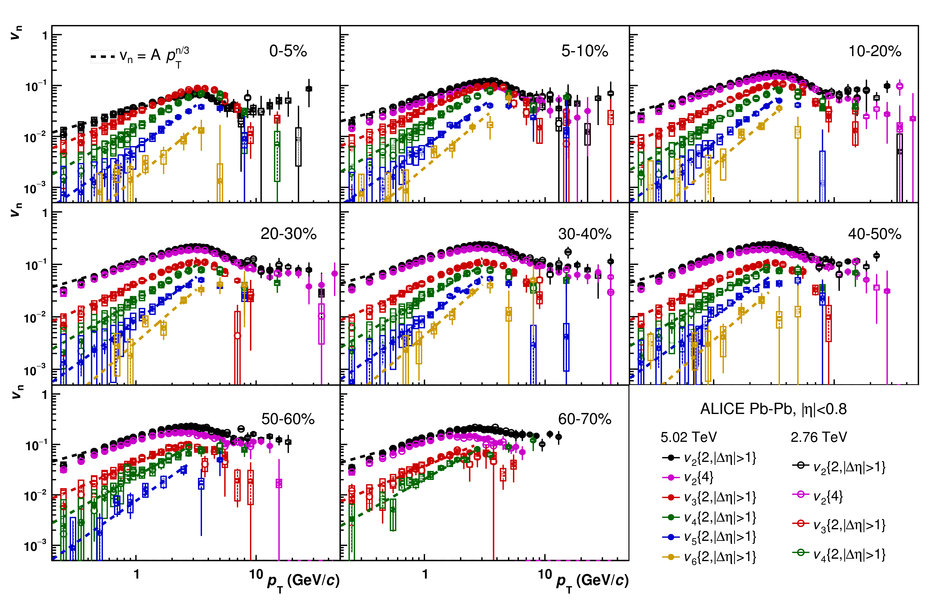Figure 4

 Anisotropic flow coefficients $v_n(p_\text{T})$ of inclusive charged particles in different centrality classes, measured with the scalar product method with respect to the V0A $Q$-vector. Measurements for Pb--Pb collisions at $\sqrt{{\textit s}_\text{NN}} = 5.02$ (2.76) TeV are shown by solid (open) markers.Figure 5

 Ratios of anisotropic flow coefficients $v_n(p_\text{T})$ of inclusive charged particles between Pb--Pb collisions at $\sqrt{{\textit s}_\text{NN}} = 5.02$ and 2.76 TeV, in different centrality classes, measured with two-particle (denoted with $|\Delta\eta|>1$) and four-particle cumulant methods.Figure 6

 Anisotropic flow coefficients $v_n(p_\text{T})$ of inclusive charged particles in different centrality classes, measured with two- and four-particle cumulant and scalar product methods with respect to the V0A $Q$-vector, for Pb--Pb collisions at $\sqrt{{\textit s}_\text{NN}} = 5.02$ TeV. Several hydrodynamic calculations and previous measurements from CMS are shown for comparison.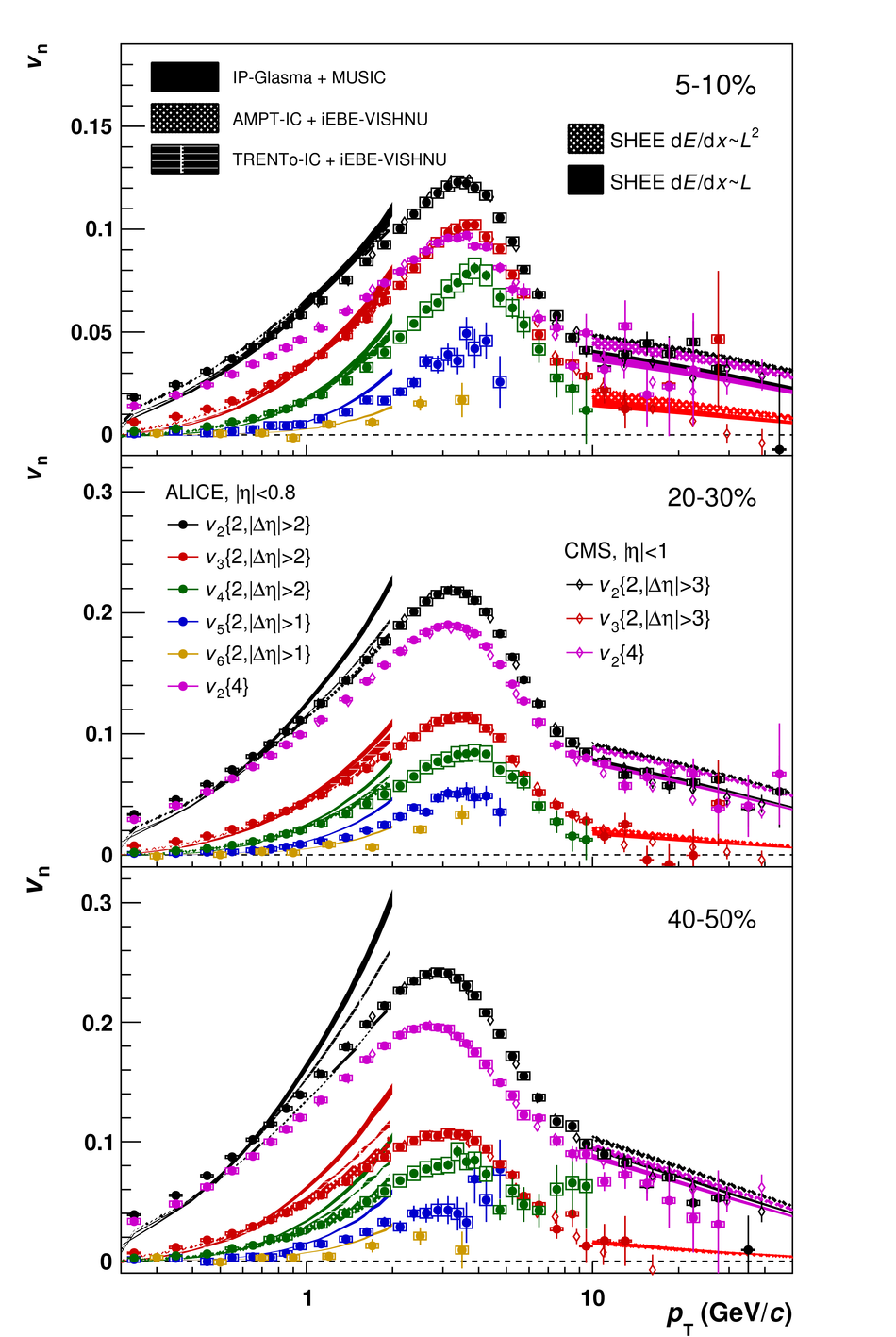Figure 7

 Ratios $v_n(p_\text{T})_\text{ratio to \text{20-30\%}}$ of inclusive charged particles for Pb--Pb collisions at $\sqrt{{\textit s}_\text{NN}} = 5.02$ TeV, in different centrality classes, measured with the scalar product method with respect to the V0A $Q$-vector. Hydrodynamic calculations are shown for comparison.Figure 8

 Ratios $v_n(p_\text{T})/v_m(p_\text{T})^{n/m}, \; n=3,4, \; m=2,3$ of inclusive charged particles for Pb--Pb collisions at $\sqrt{{\textit s}_\text{NN}} = 5.02$, in different centrality classes, measured with the scalar product method with respect to the V0A $Q$-vector. Dashed lines represent averages in $0.2 < p_\text{T} < 3$ GeV/$c$. The ratios are also shown for one hydrodynamic model in the four most central centrality intervals; it is qualitatively similar in the other centrality intervals and for the other models.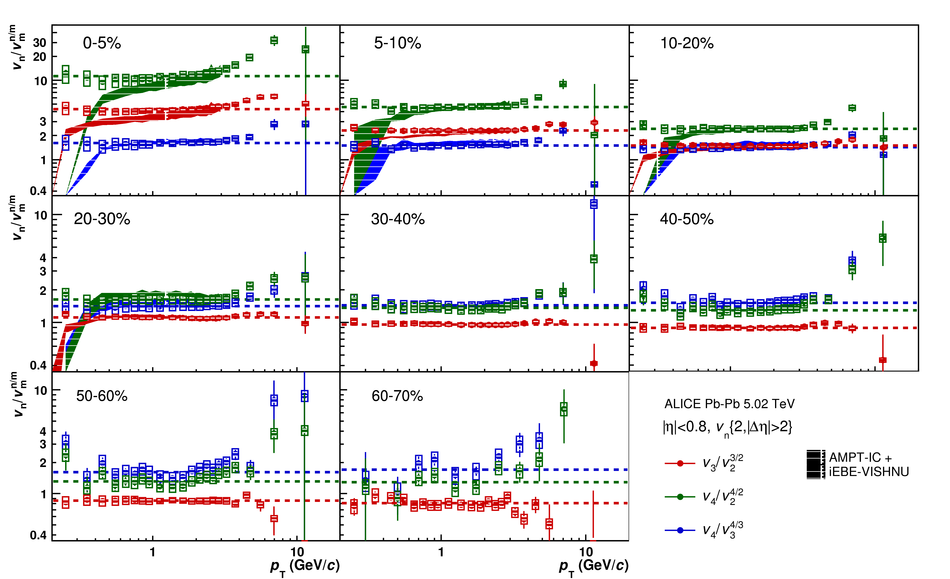Figure 9

 Elliptic flow coefficient $v_2$ of inclusive charged particles as a function of centrality, measured with the two- and multi-particle cumulant methods. Measurements for Pb--Pb collisions at $\sqrt{{\textit s}_\text{NN}} = 5.02$ (2.76) TeV are shown by solid (open) markers.Figure 10

 Cumulants $c_2$ of elliptic flow of inclusive charged particles as a function of centrality, for the two-particle and multi-particle cumulant methods. Measurements for Pb--Pb collisions at $\sqrt{{\textit s}_\text{NN}} = 5.02$ (2.76) TeV are shown by solid (open) markers.Figure 11

 Ratios of elliptic flow coefficients $v_2$ of inclusive charged particles between measurements with different multi-particle cumulant methods, as a function of centrality. Measurements at $\sqrt{{\textit s}_\text{NN}} = 5.02$ (2.76) TeV are shown by solid (open) markers.Figure 12

 Ratios of elliptic flow coefficients $v_2$ of inclusive charged particles between measurements with different multi-particle cumulant methods, as a function of centrality, at $\sqrt{{\textit s}_\text{NN}} = 2.76$ TeV. Hydrodynamic calculations and ATLAS measurements are shown for comparison.Figure 13

 Ratio of elliptic flow coefficients $v_2\{8\}/v_2\{6\}$ of inclusive charged particles as a function of centrality.Figure 14

 Differences of elliptic flow coefficients $v_2$ of inclusive charged particles between measurements with different multi-particle cumulant methods, as a function of centrality.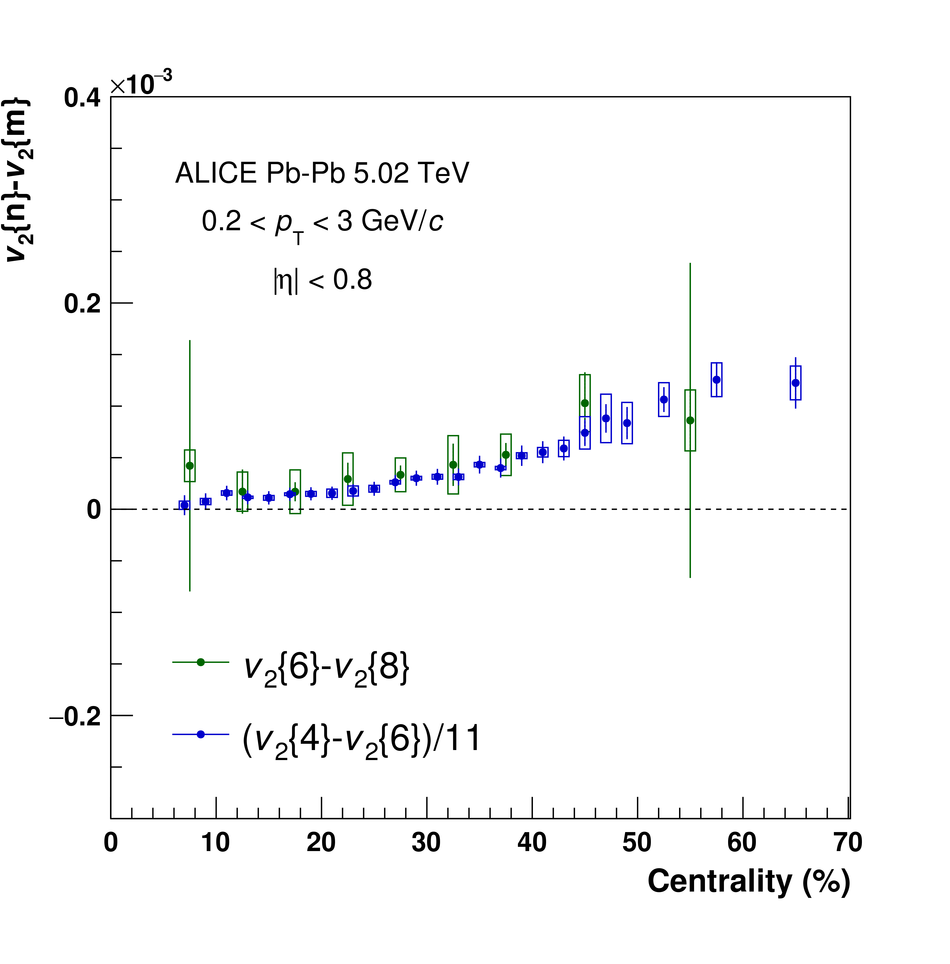Figure 15

 Skewness of elliptic flow $\gamma_1^\text{exp}$ of inclusive charged particles as a function of centrality, for Pb--Pb collisions at $\sqrt{{\textit s}_\text{NN}} = 5.02$ TeV. Hydrodynamic calculations for Pb--Pb collisions at $\sqrt{{\textit s}_\text{NN}} = 2.76$ TeV are shown for comparison.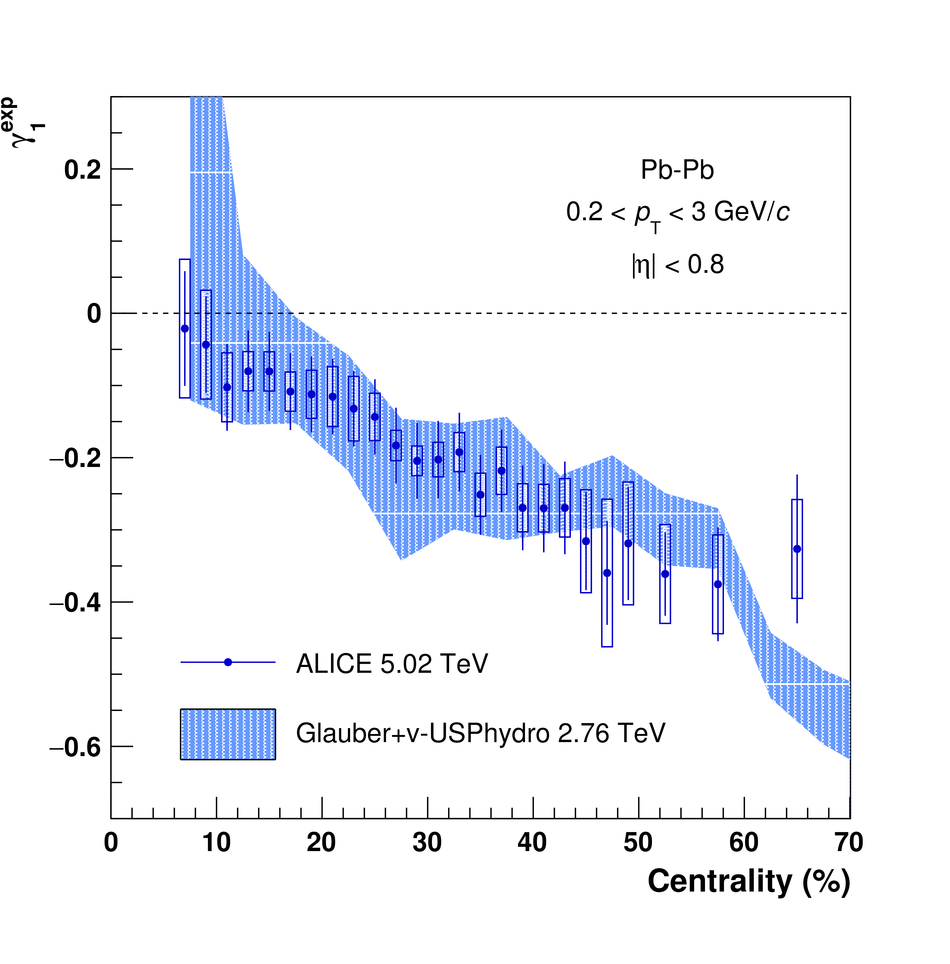Figure 16

 Elliptic power parameters $k_2$, $\alpha$ and $\varepsilon_0$ as a function of centrality, for Pb--Pb collisions at $\sqrt{{\textit s}_\text{NN}} = 5.02$ TeV, extracted from measurements of $v_2$ of inclusive charged particles with two-particle and multi-particle cumulant methods.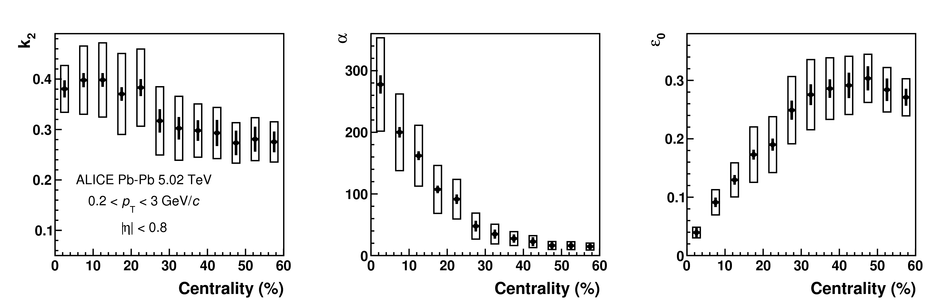Figure 17

 Elliptic flow p.d.f. $P(v_2)$ rescaled by the mean $v_2$ ($\langle v_2 \rangle$) of inclusive charged particles for Pb--Pb collisions at $\sqrt{{\textit s}_\text{NN}} = 5.02$ TeV, in different centrality classes. Several hydrodynamic calculations and previous measurements from ATLAS at lower energies are shown for comparison.Figure 18

 Elliptic flow p.d.f. $P(v_2)$ rescaled by $\langle v_2 \rangle$ in centralities 0--5\%, 10--15\% and 15--20\% for Pb--Pb collisions at $\sqrt{{\textit s}_\text{NN}} = 5.02$ TeV.Figure 19

 Elliptic flow p.d.f. $P(v_2)$ rescaled by $\langle v_2 \rangle$ in centralities 20--25\%, 30--35\% and 35--40\% for Pb--Pb collisions at $\sqrt{{\textit s}_\text{NN}} = 5.02$ TeV.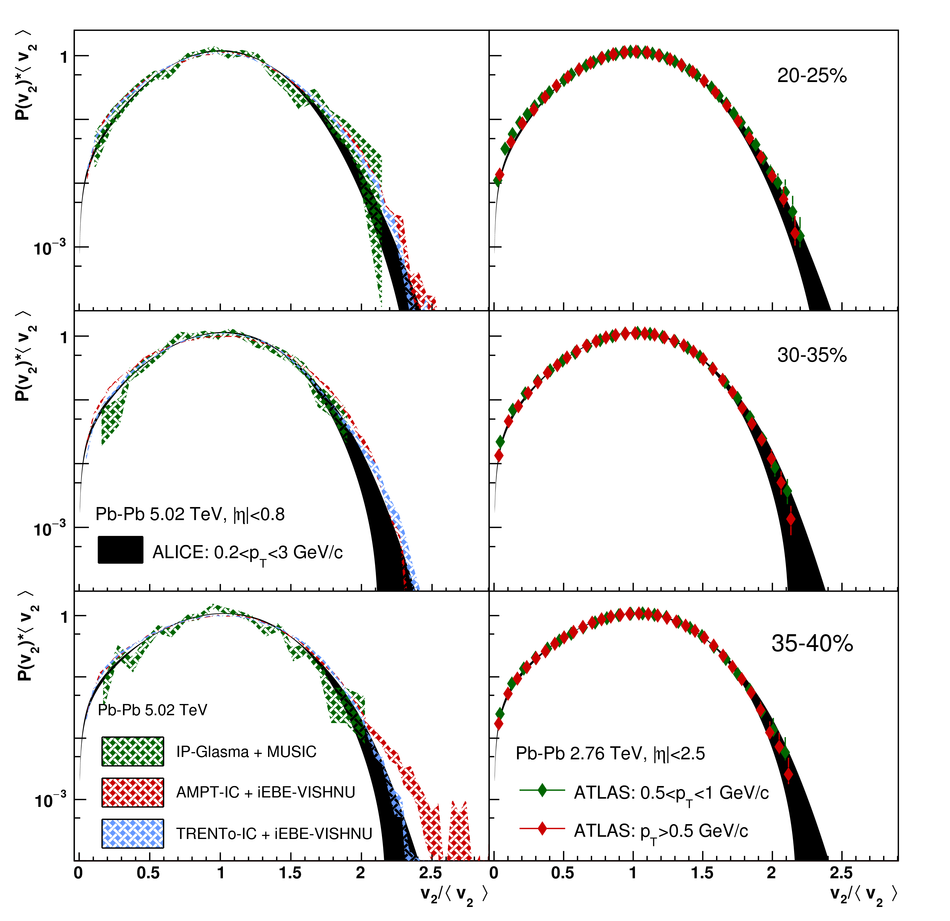Figure 20

 Elliptic flow p.d.f. $P(v_2)$ rescaled by $\langle v_2 \rangle$ in centralities 40--45\%, 50--55\% and 55--60\% for Pb--Pb collisions at $\sqrt{{\textit s}_\text{NN}} = 5.02$ TeV.